# ZONK Algebra 1 Midterm Review ZONK directions 1

• Slides: 49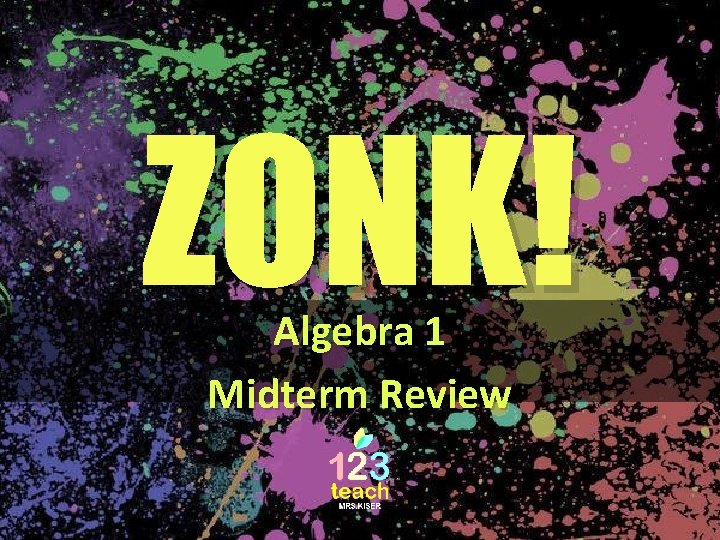ZONK! Algebra 1 Midterm Review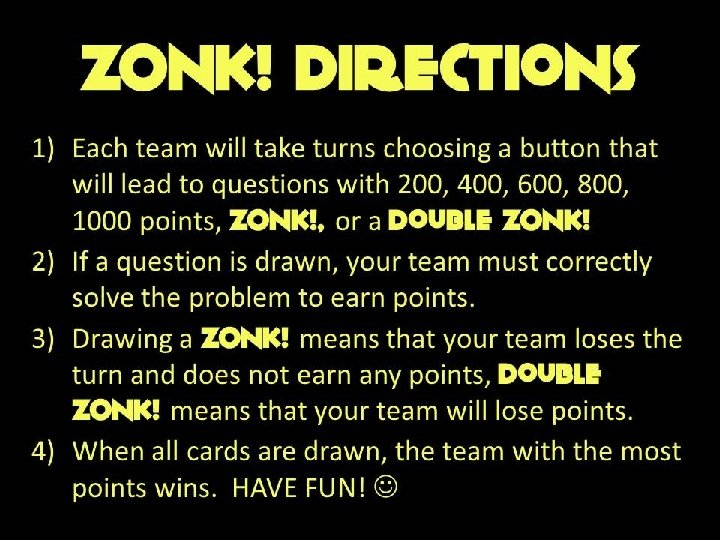ZONK! directions 1) Each team will take turns choosing a button that will lead to questions with 200, 400, 600, 800, 1000 points, ZONK!, or a Double ZONK! 2) If a question is drawn, your team must correctly solve the problem to earn points. 3) Drawing a ZONK! means that your team loses the turn and does not earn any points, Double ZONK! means that your team will lose points. 4) When all cards are drawn, the team with the most points wins. HAVE FUN!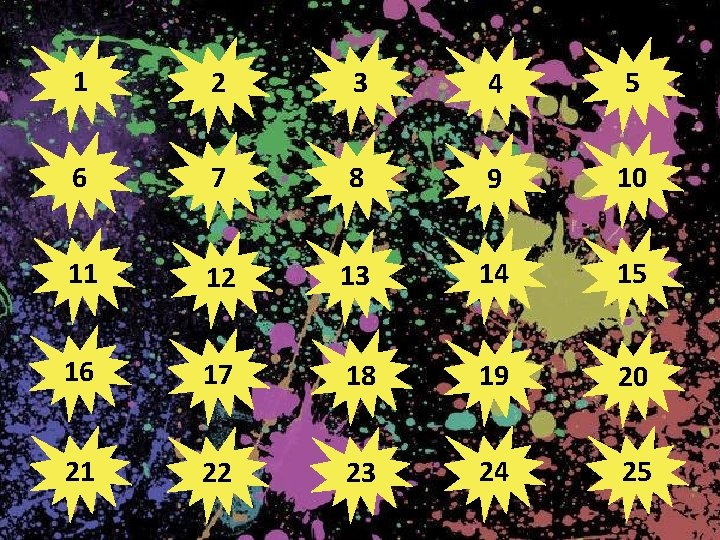1 2 3 4 5 6 7 8 9 10 11 12 13 14 15 16 17 18 19 20 21 22 23 24 25GREAT JOB! Thank you for playing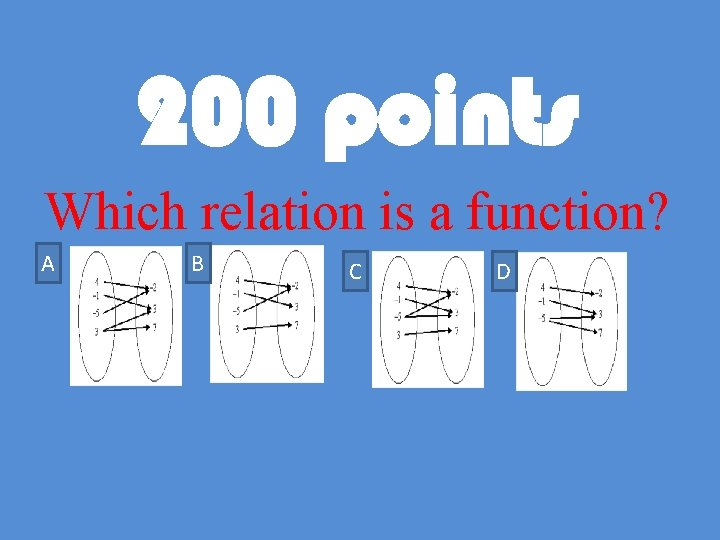200 points Which relation is a function? A B C D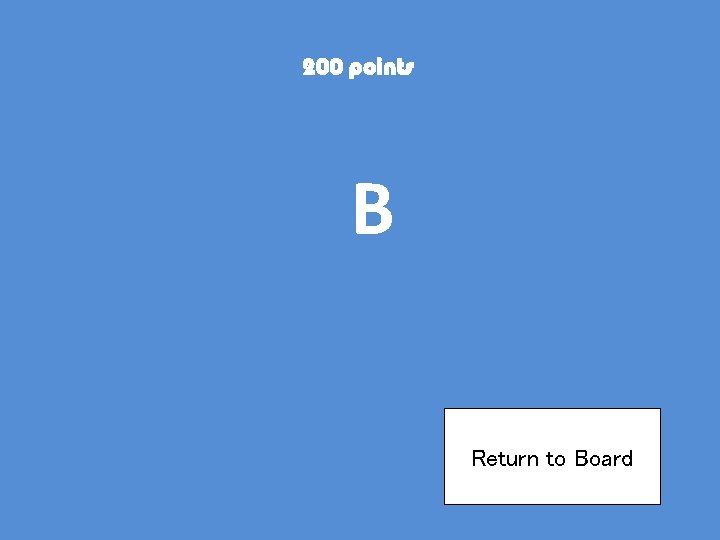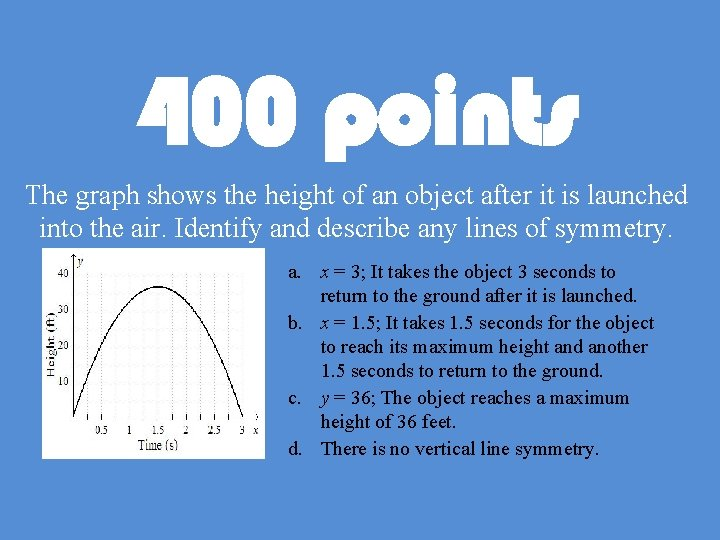400 points The graph shows the height of an object after it is launched into the air. Identify and describe any lines of symmetry. a. x = 3; It takes the object 3 seconds to return to the ground after it is launched. b. x = 1. 5; It takes 1. 5 seconds for the object to reach its maximum height and another 1. 5 seconds to return to the ground. c. y = 36; The object reaches a maximum height of 36 feet. d. There is no vertical line symmetry.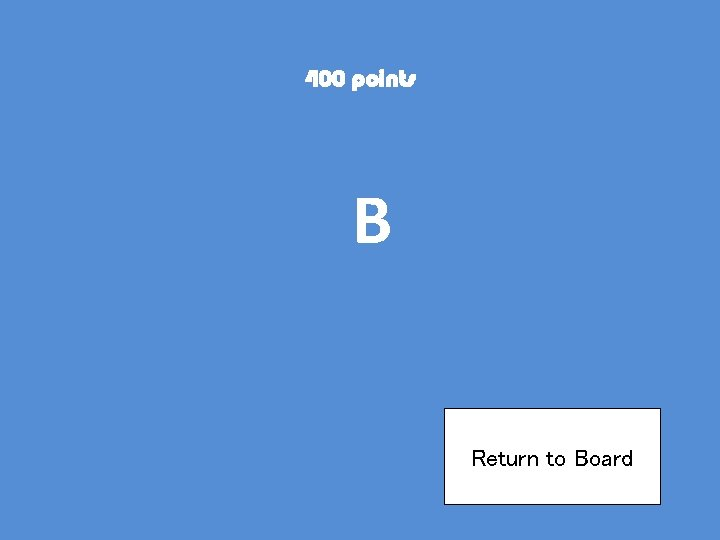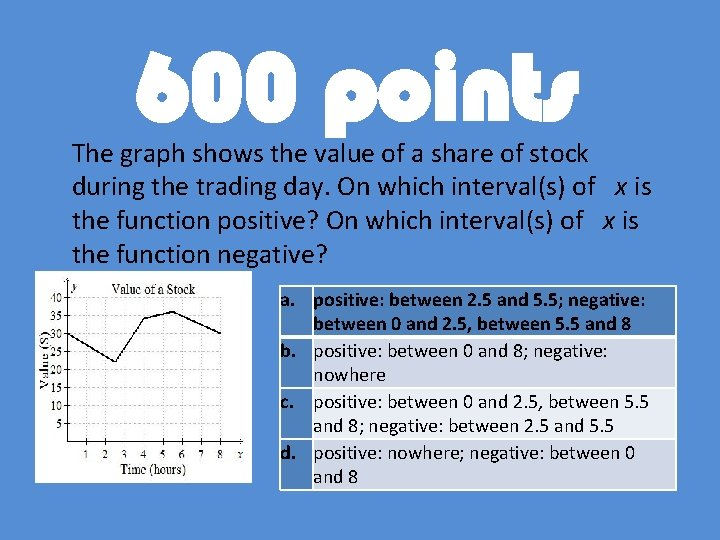600 points The graph shows the value of a share of stock during the trading day. On which interval(s) of x is the function positive? On which interval(s) of x is the function negative? a. positive: between 2. 5 and 5. 5; negative: between 0 and 2. 5, between 5. 5 and 8 b. positive: between 0 and 8; negative: nowhere c. positive: between 0 and 2. 5, between 5. 5 and 8; negative: between 2. 5 and 5. 5 d. positive: nowhere; negative: between 0 and 8800 points Find three consecutive even integers with a sum of 48.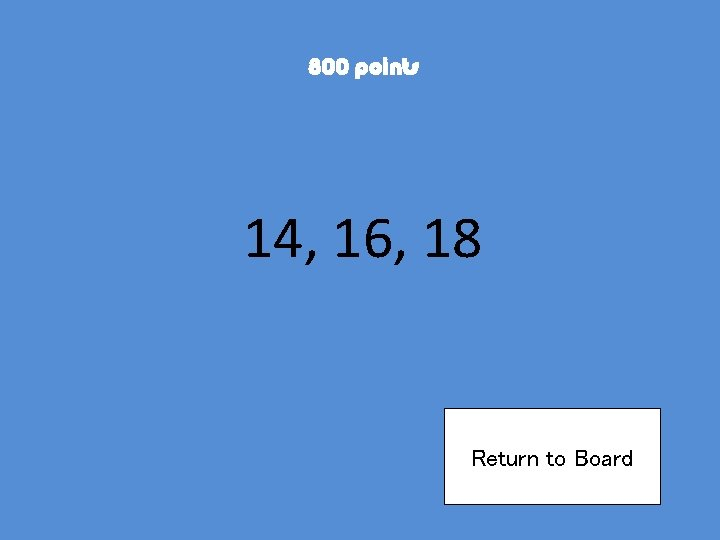1000 points Solve the equation – 16(10 u – 90) = 32(8 u + 71)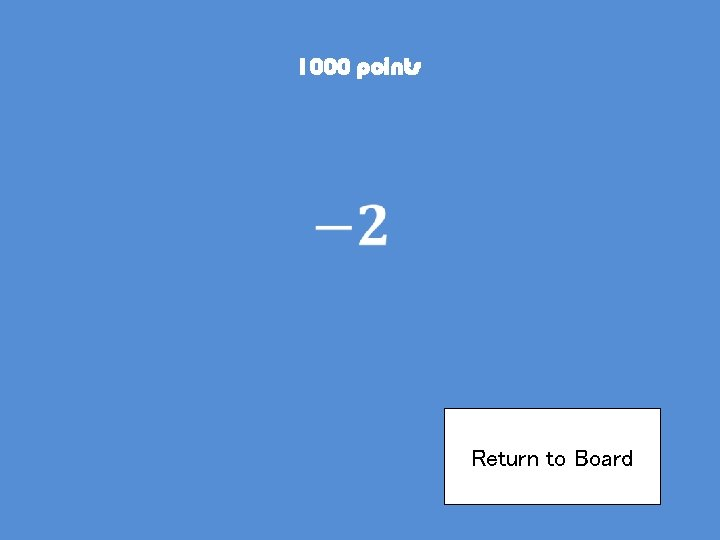200 points Find the slope of the line that passes through the pair of points. Show all your work in solving. (– 4, 1), (5, 4)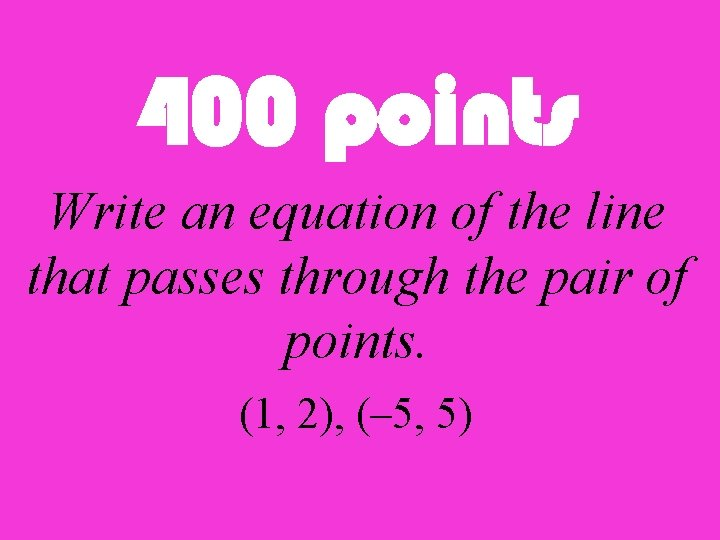400 points Write an equation of the line that passes through the pair of points. (1, 2), (– 5, 5)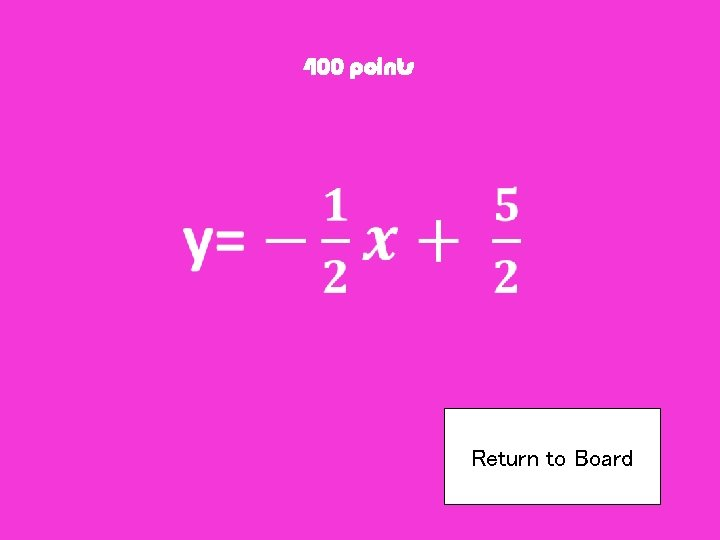600 points Write the slope-intercept form of an equation of the line that passes through the given point and is parallel to the graph of the equation. (4, 1), x + 4 y = – 12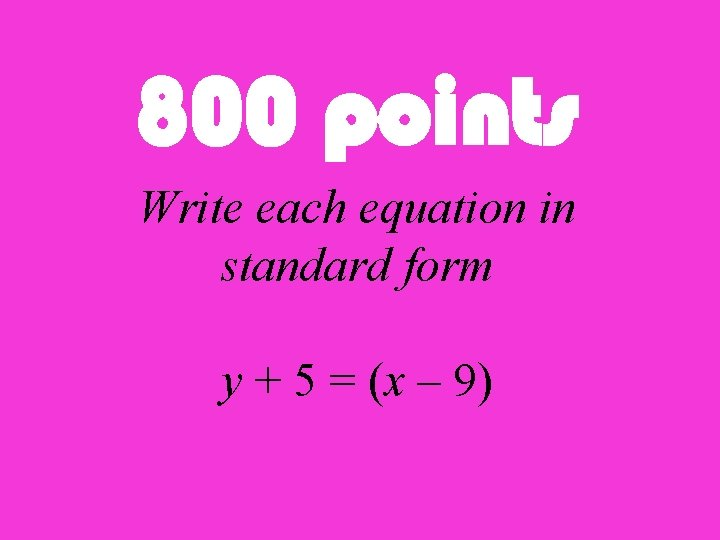800 points Write each equation in standard form y + 5 = (x – 9)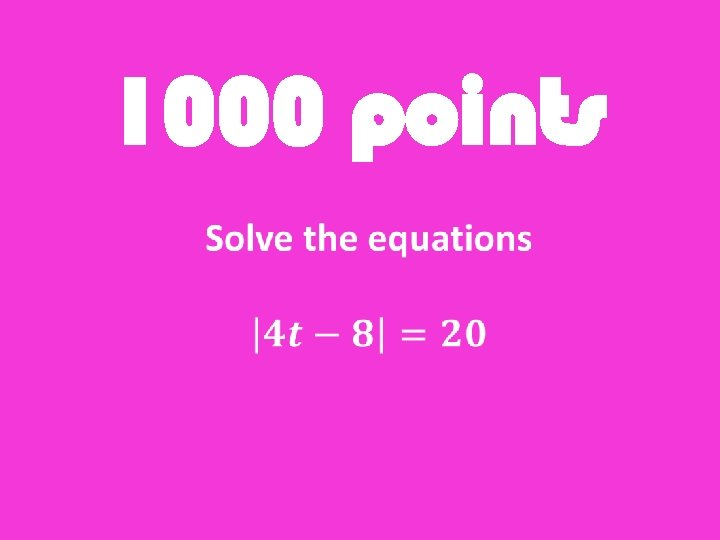1000 points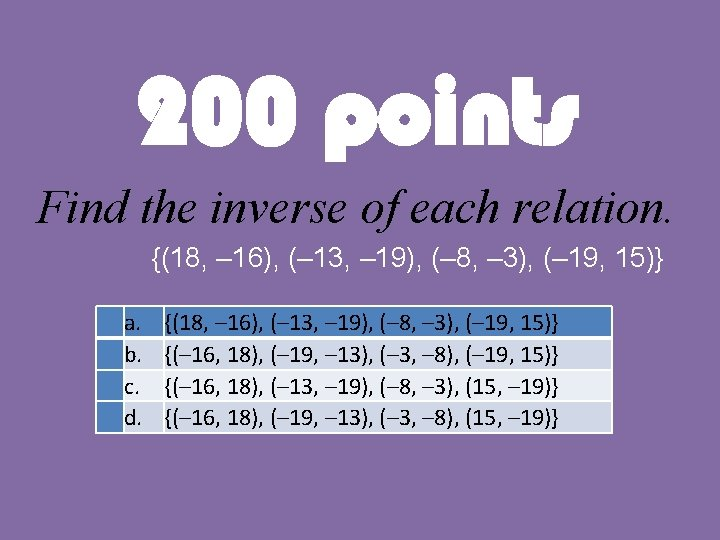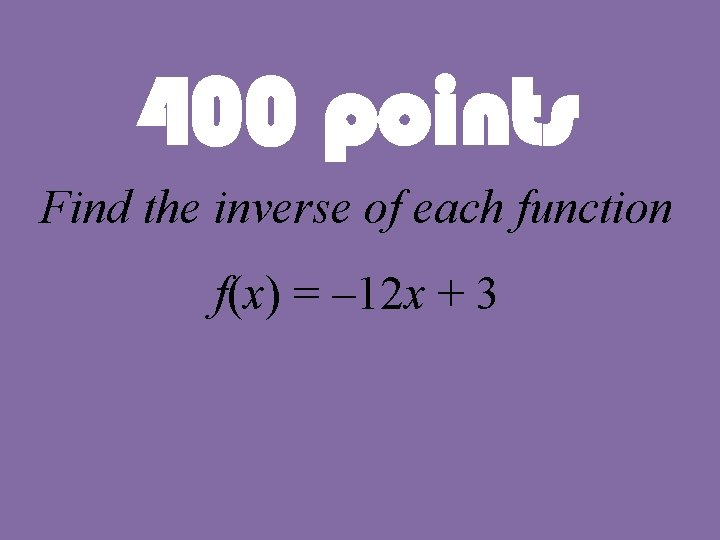400 points Find the inverse of each function f(x) = – 12 x + 3600 points Solve the inequality. 4. 6 s – 3. 2 ≤ 2. 5 s – 1. 52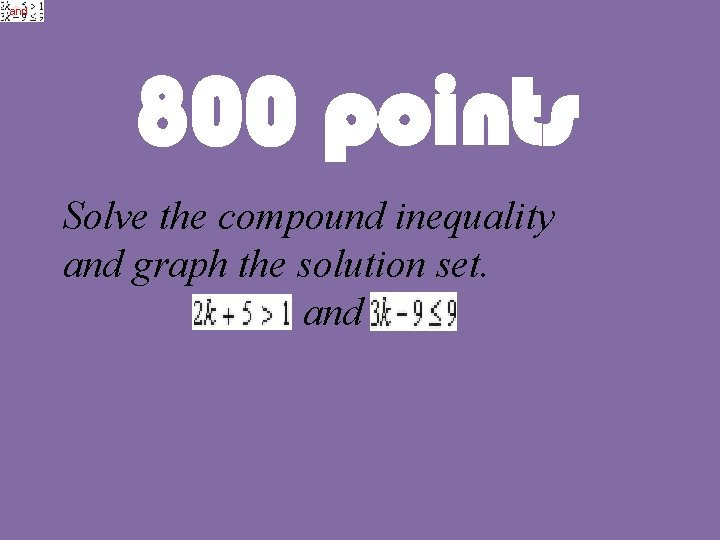and 800 points Solve the compound inequality and graph the solution set. and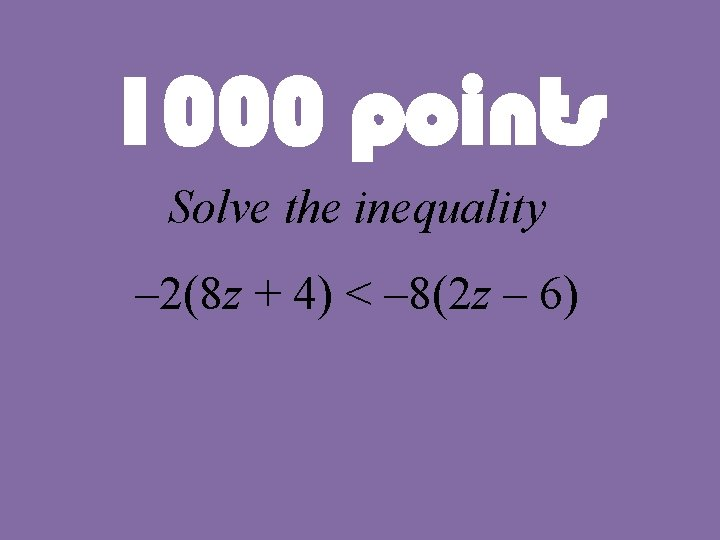1000 points Solve the inequality – 2(8 z + 4) < – 8(2 z – 6)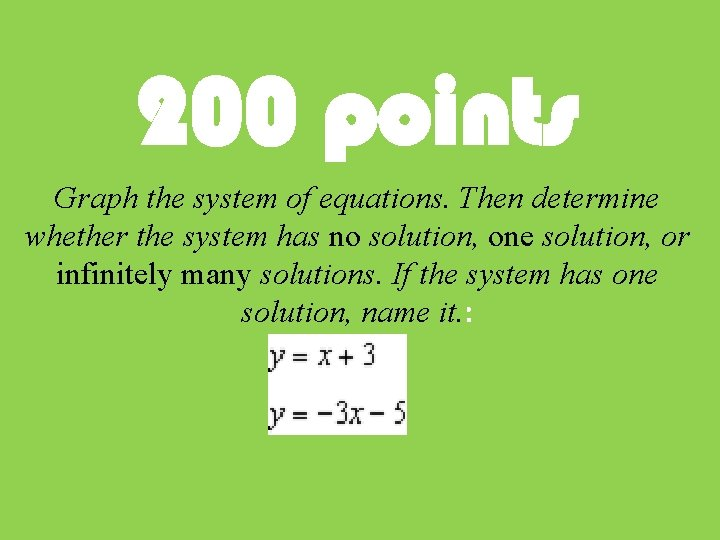200 points Graph the system of equations. Then determine whether the system has no solution, one solution, or infinitely many solutions. If the system has one solution, name it. :400 points Use substitution to solve the system of equations y = – 3 x + 27 8 x – 3 y = 123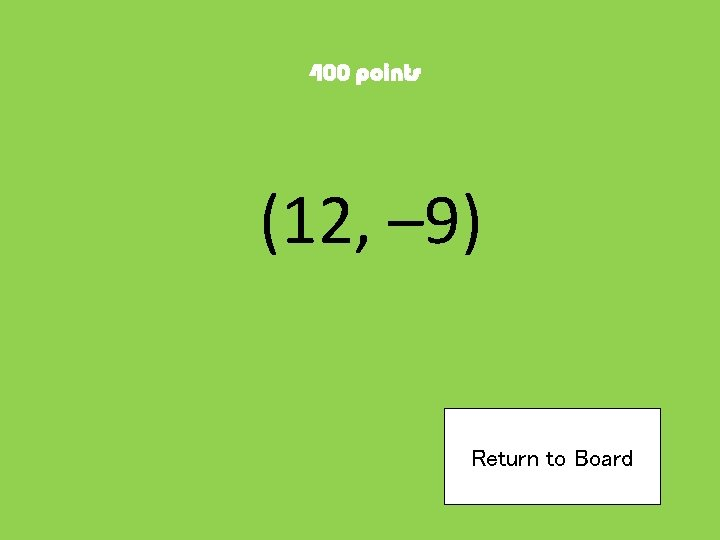600 points Use elimination to solve the system of equations. 6 x – 8 y = – 54 – 3 x + 12 y = 99800 points. Joji earns 3 times as much as Masao. If Joji and Masao earn \$4500. 00 together, how much money does Masao earn?1000 points